# 7-图¶

• 线性表的数据元素 -> 元素 Element
• 树中的数据元素 -> 结点 Node
• 图中的数据元素 -> 顶点 Vertex1

• 线性表没有数据元素 -> 空表

• 树中没有数据元素 -> 空树
• 图中没有数据元素 -> 不行！

## 定义¶

### 无向边¶### 有向边¶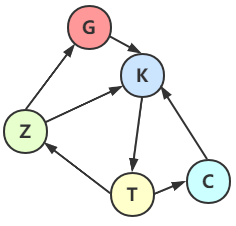Warning

### 简单图¶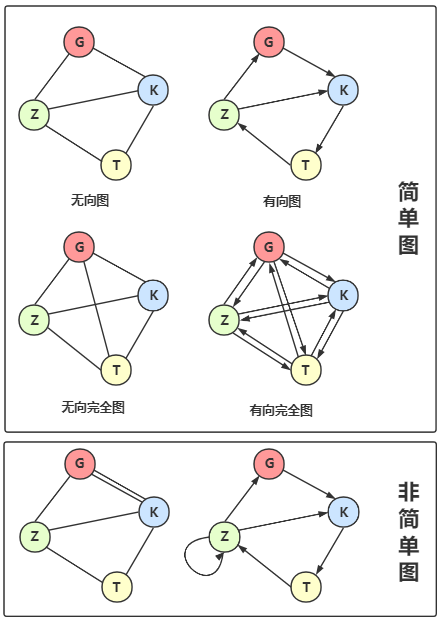Tip

### 权、网¶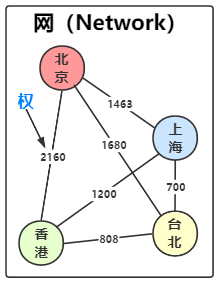### 子图¶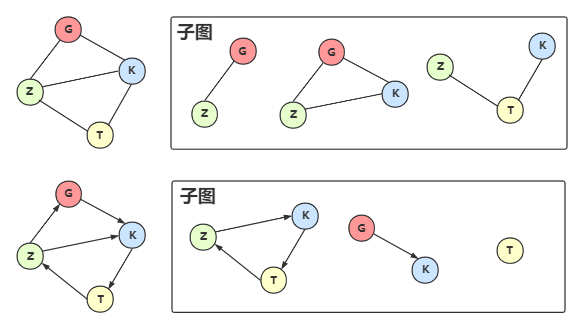## 图的顶点和边间的关系¶

### 度¶

#### 无向图的度¶

• 如果边 $$(v, v') \in E$$，则称顶点 $$v$$$$v'$$ 互为 邻接点（Adjacent），即 $$v$$$$v'$$ 相邻接。
• $$(v, v')$$ 依附（Incident） 于顶点 $$v$$$$v'$$，即边 $$(v, v')$$ 与顶点 $$v$$$$v'$$ 相关联。
• 顶点 $$v$$度（Degree）12 是和 $$v$$ 相关的边的数量，记为 $$TD(v)$$

eg: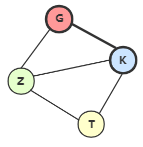$e= \frac 1 2 \sum_{i=1}^n TD(v_i)$

#### 有向图的度¶

• 如果弧 $$\langle v, v' \rangle \in E$$，则称：顶点 $$v$$ 邻接到 $$v'$$，顶点 $$v'$$ 邻接自 $$v$$。顶点 $$v$$$$v'$$ 互为 邻接点（Adjacent）
• $$\langle v, v' \rangle$$ 依附（incident） 于顶点 $$v$$$$v'$$，即弧 $$\langle v, v' \rangle$$ 与 顶点 $$v$$$$v'$$ 相关联。
• 以顶点 $$v$$ 为头的弧的数量称为 $$v$$入度（InDegree）13，记为 $$ID(v)$$;
• 以顶点 $$v$$ 为尾的弧的数量称为 $$v$$出度（OutDegree）14，记为 $$OD(v)$$;
• 顶点 $$v$$度（Degree）$$TD(v) = ID(v) + OD(v)$$

$$(G, K)$$ 依附于 $$G$$$$K$$ 上，顶点 $$K$$ 的入度为 2，$$ID(K) = 2$$，出度为 1，$$OD(K) = 1$$，度为 $$TD(K) = ID(K) + OD(K) = 3$$

$e= \sum_{i=1}^n ID(V_i) = \sum_{i=1}^n OD(v_i)$

### 路径¶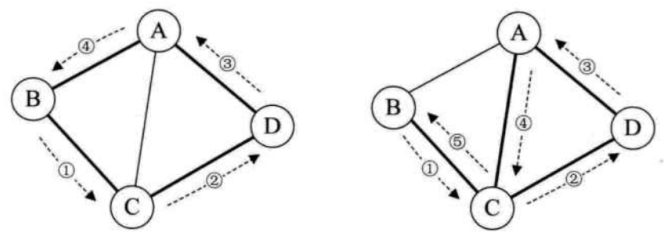## 连通图¶

### 无向连通图¶

• 必须是子图（子图
• 子图是连通的（连通
• 连通子图含有极大顶点数（有极大顶点数
• 具有极大顶点数的连通子图包含依附于这些顶点的所有边。（含有边

### 生成树、生成森林¶

#### 生成树¶

NoteNote

1. 包含连通图中所有的顶点；
2. 任意两顶点之间有且仅有一条通路；

$\begin{cases} 非连通图, & 顶点个数 = n;边数 \lt n-1 \\\\ 生成树, & 顶点个数 = n;边数 = n-1 \\\\ 一定有环, & 顶点个数 = n;边数 \gt n-1 \\\\ \end{cases}$

#### 生成森林¶

Note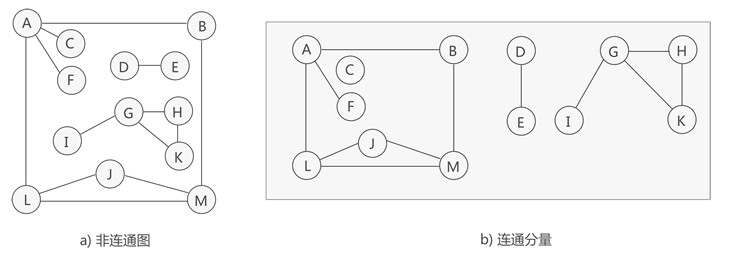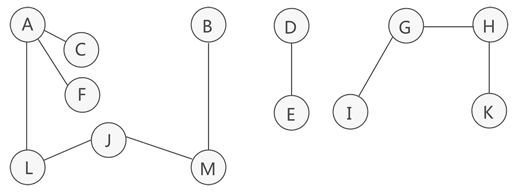上图只是列出各个连通分量的其中一种生成树。

## 总结¶

• 图由顶点和边组成，无向图由 顶点 和 边 构成有向图由 顶点 和 弧 构成，弧分 弧头弧尾
• 任两个顶点之间都存在边叫 完全图，分 有向完全图无向完全图。同一条边不重复出现的图叫 简单图
• 顶点之间有 邻接点依附 的概念，无向图的顶点的边数叫 度有向图的顶点分 出度 和 入度 ，度 = 出+入
• 从图的顶点集和边集中取一小部分组成新的图，称为原图的 子图
• 带数值的边叫 ，顶点和权构成
• 图中顶点间存在 路径，两顶点存在路径则说明是 连通 的，如果路径最终回到起始点则称为 ，当中顶点没有重复过称为 简单路径。若任意两顶点都是连通的，则图是 连通图，有向则是 强连通图。图中有子图，若子图极大连通则称 连通分量，有向则称 强连通分量
• 无向图中连通且边数 = 顶点数 - 1 的树叫 生成树，无向非连通图的连通分量生成的生成树一起构成 生成森林
• 有向图中一个顶点入度为 0，其他顶点的入度为1的叫 有向树。一个有向图由若干棵有向树构成生成森林。

1. 顶点 -> 图的数据元素

2. 无向边 -> 边没有方向

3. 无向图 -> 边没有方向的图，n个顶点的无向图最多有 $$\frac {n(n-1)} 2$$ 条边

4. 弧 -> 边有方向；弧有弧头弧尾之分

5. 有向图 -> 边有方向的图，n个顶点的有向图最多有 $$n(n-1)$$ 条边

6. 简单图 -> 同一条边不重复出现的图

7. 无向完全图 -> 任意两个顶点之间都有边，共有 $$\frac {n(n-1)} 2$$ 条边

8. 有向完全图 -> 任意两个顶点之间都有弧，共有 $$n(n-1)$$ 条弧

9. 权 -> 有数值的边

10. 网 -> 顶点+权

11. 子图 -> 从原图中拆出来的图

12. 度 ->顶点的边数

13. 入度 -> 邻接到顶点的边的数量

14. 出度 -> 顶点邻接出去的边的数量

15. 路径 -> 两个顶点之间的直接线路或间接线路的边集序列，注意是序列，所以可能不止一条

16. 路径长度 -> 两个顶点之间的线路的边数

17. 回路或环 -> 从起始点离开最后到达起始点的路径

18. 简单路径 -> 回路上没有重复的点的路径

19. 连通图 -> 任意两顶点都是连通的无向图

20. 连通分量 -> 无向图的极大连通的子图

21. 强连通图 -> 任意两顶点都是连通的有向图

22. 强连通分量 -> 有向图的极大连通的子图

23. 生成树 -> 无向连通图生成的 边的数量 = 顶点数 -1 的树

24. 生成森林 -> 无向非连通图的连通分量生成的生成树一起构成生成森林